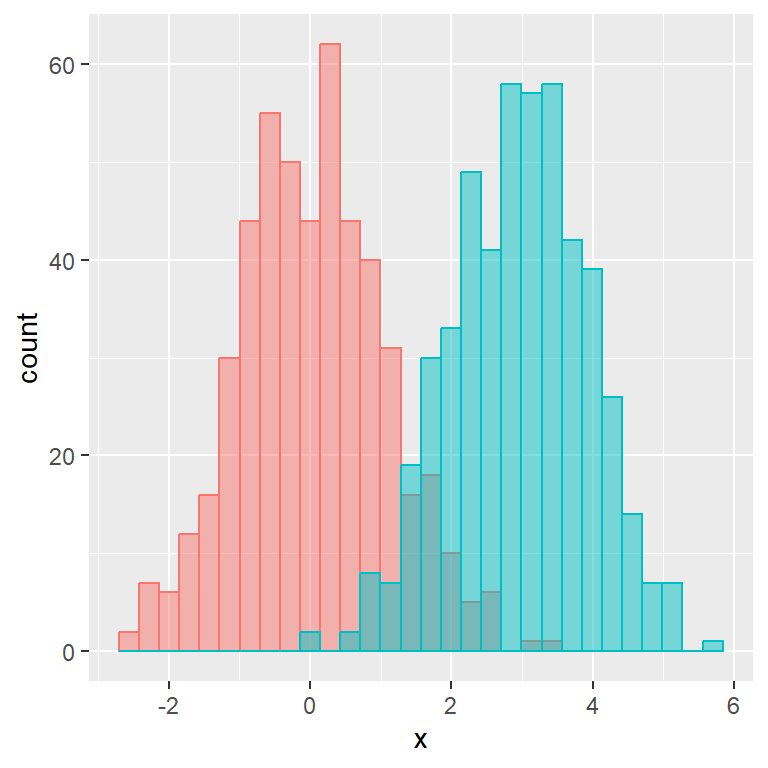# Histogram by group in ggplot2

## Sample data

The following data frame contains a column with two normal distributions with different mean and same variance and a categorical variable representing which observations belong to each distribution.

``````set.seed(3)
x1 <- rnorm(500)
x2 <- rnorm(500, mean = 3)
x <- c(x1, x2)
group <- c(rep("G1", 500), rep("G2", 500))

df <- data.frame(x, group = group)``````

## Grouped histogram with `geom_histogram`

Fill

In order to create a histogram by group in ggplot2 you will need to input the numerical and the categorical variable inside `aes` and use `geom_histogram` as follows.

``````# install.packages("ggplot2")
library(ggplot2)

# Histogram by group in ggplot2
ggplot(df, aes(x = x, fill = group)) +
geom_histogram()``````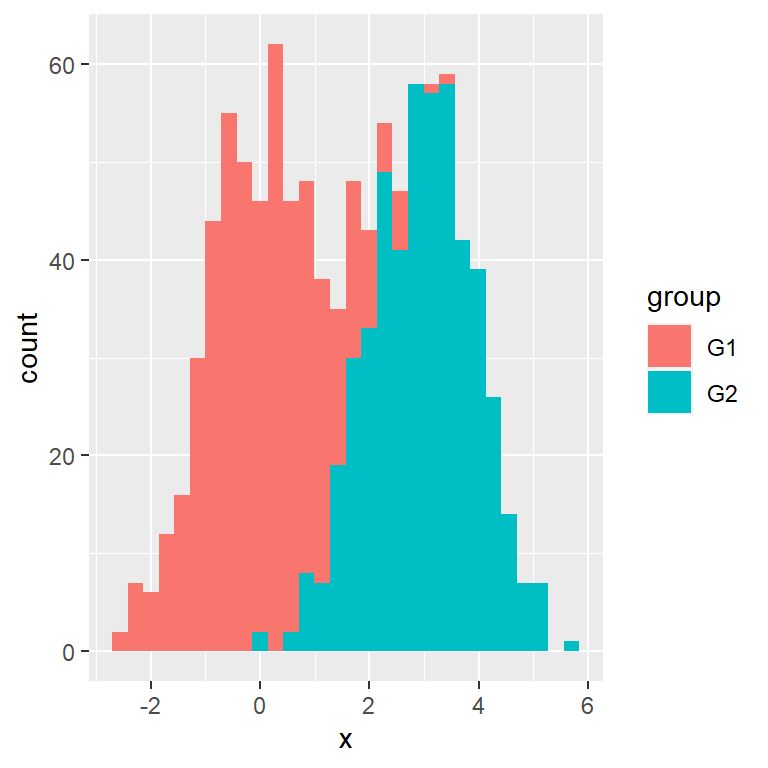Colour

You can also set the categorical variable to the `colour` argument, so the border lines of each histogram will have a different color.

``````# install.packages("ggplot2")
library(ggplot2)

# Histogram by group in ggplot2
ggplot(df, aes(x = x, colour = group)) +
geom_histogram()``````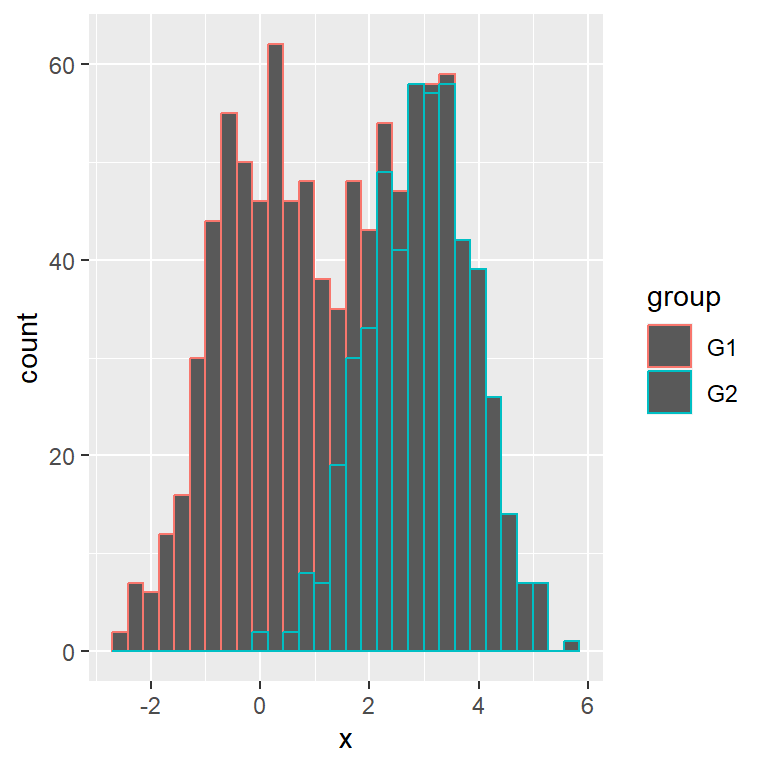By default, if the histograms overlap, the values will be stacked. Another approach is changing the position to `identity` (and setting transparency) or `dodge` as in the following examples.

identity position

Setting `position = "identity"` is the most common use case, but recall to set a level of transparency with `alpha` so both histograms are completely visible.

``````# install.packages("ggplot2")
library(ggplot2)

# Histogram by group in ggplot2
ggplot(df, aes(x = x, fill = group, colour = group)) +
geom_histogram(alpha = 0.5, position = "identity")``````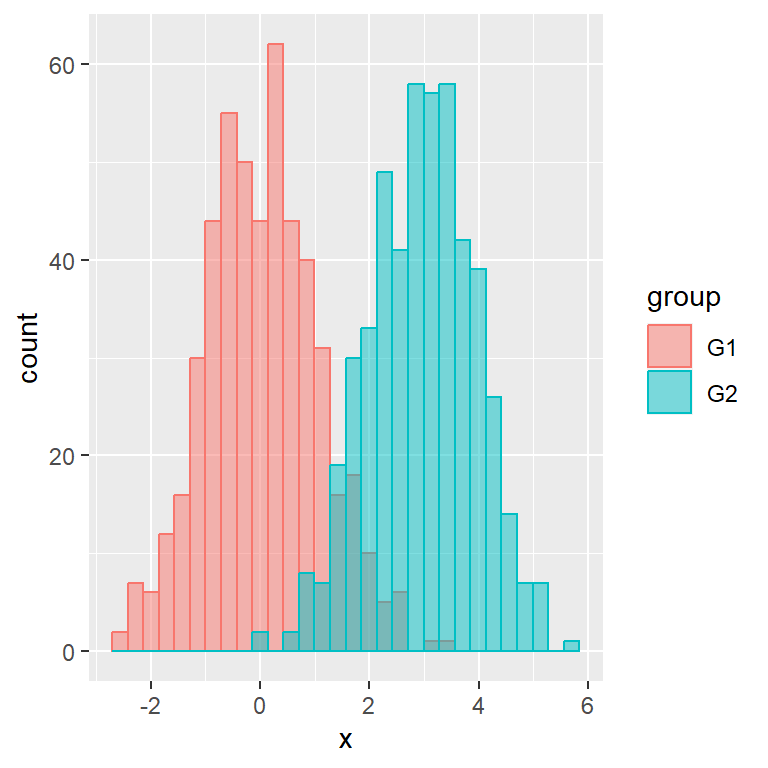dodge position

Other option is using `position = "dodge"`, which will add an space between each bar so you will be able to see both histograms.

``````# install.packages("ggplot2")
library(ggplot2)

# Histogram by group in ggplot2
ggplot(df, aes(x = x, fill = group, colour = group)) +
geom_histogram(position = "dodge")``````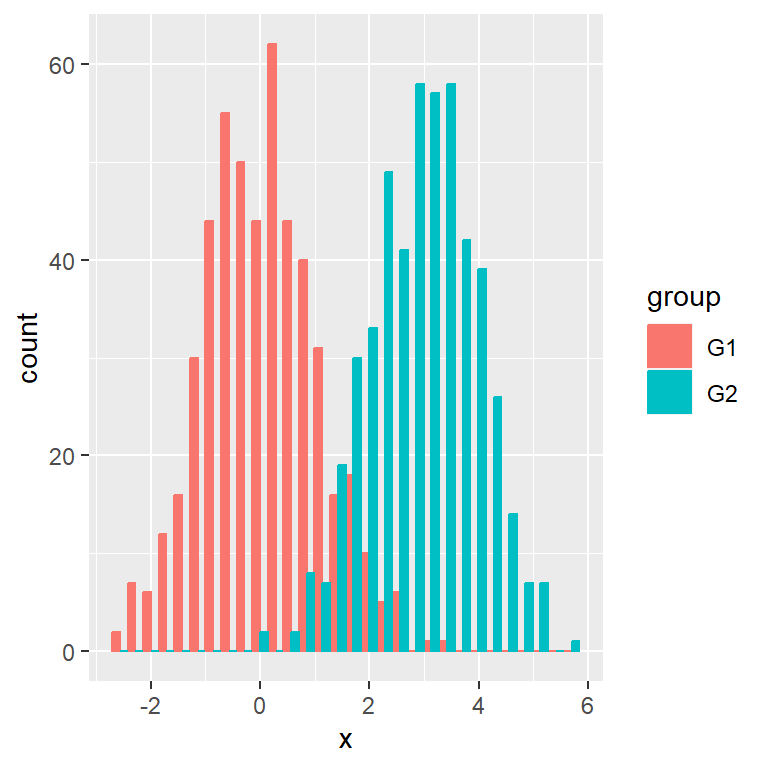## Histogram by group with custom colors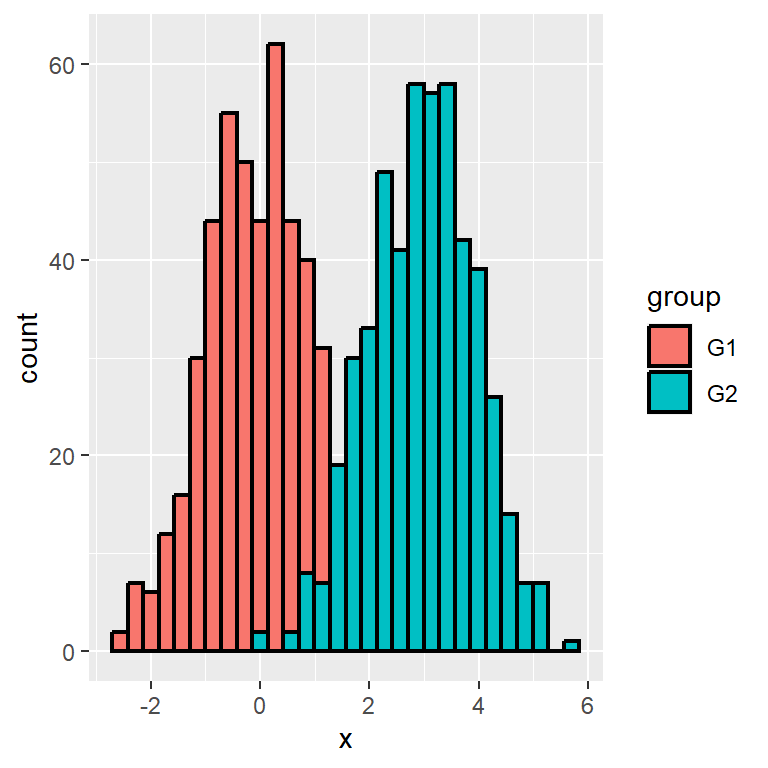Borders color

If you set `fill` inside `aes` but not `colour` you can change the border color of all histograms as well as its width and linetype with `geom_histogram` arguments.

``````# install.packages("ggplot2")
library(ggplot2)

# Histogram by group in ggplot2
ggplot(df, aes(x = x, fill = group)) +
geom_histogram(colour = "black",
lwd = 0.75,
linetype = 1,
position = "identity")``````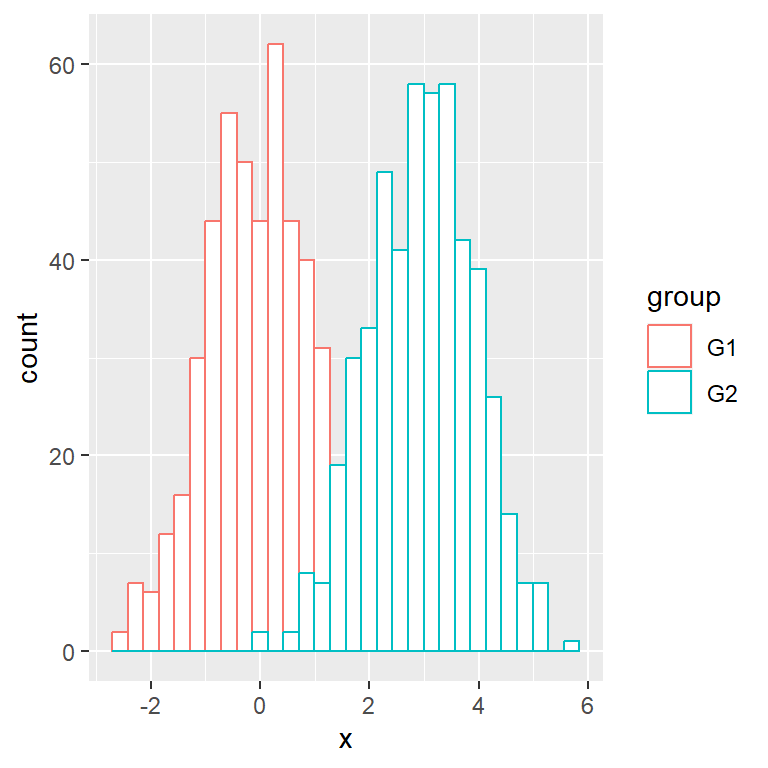Fill color

If you set `colour` but not `fill` you can change the fill color of all histograms with the `fill` argument of `geom_histogram`.

``````# install.packages("ggplot2")
library(ggplot2)

# Histogram by group in ggplot2
ggplot(df, aes(x = x, colour = group)) +
geom_histogram(fill  = "white",
position = "identity")``````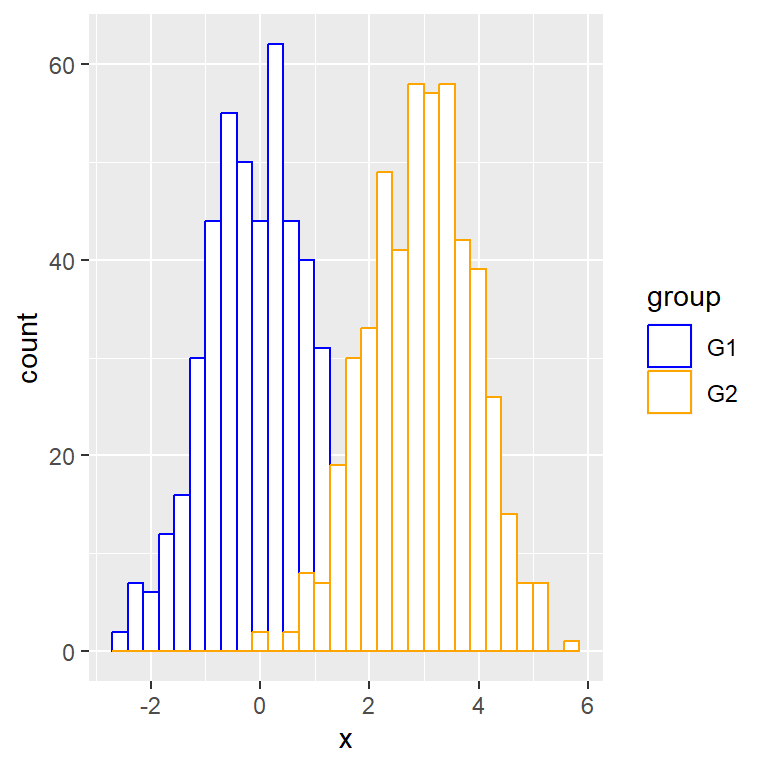Custom border colors for each group

The borders color can be customized individually with `scale_color_manual`. If you want to use a palette you can use `scale_color_brewer`, for instance.

``````# install.packages("ggplot2")
library(ggplot2)

# Histogram by group in ggplot2
ggplot(df, aes(x = x, colour = group)) +
geom_histogram(fill  = "white",
position = "identity") +
scale_color_manual(values = c("blue", "orange"))``````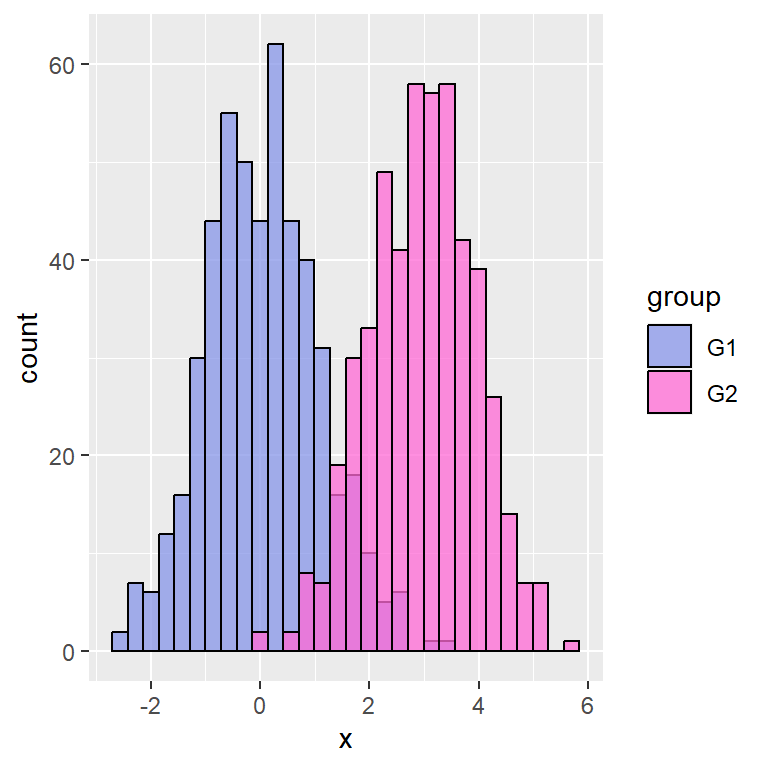Custom fill colors for each group

Similarly to customizing the borders color, the fill colors can be set with `scale_fill_manual` or any function supporting fills.

``````# install.packages("ggplot2")
library(ggplot2)

# Histogram by group in ggplot2
ggplot(df, aes(x = x, fill = group)) +
geom_histogram(color = 1, alpha = 0.75,
position = "identity") +

## Legend customization

Custom legend title

The legend title is the name of the column of the categorical value of the data set. You can change it with the `fill` and/or `colour` arguments of the `guides` function. As we are passing `fill` and `colour` to `aes` we are setting both or two legends will be displayed.

``````# install.packages("ggplot2")
library(ggplot2)

# Histogram by group in ggplot2
ggplot(df, aes(x = x, fill = group, colour = group)) +
geom_histogram(alpha = 0.5, position = "identity") +
guides(fill = guide_legend(title = "Title"),
colour = guide_legend(title = "Title"))``````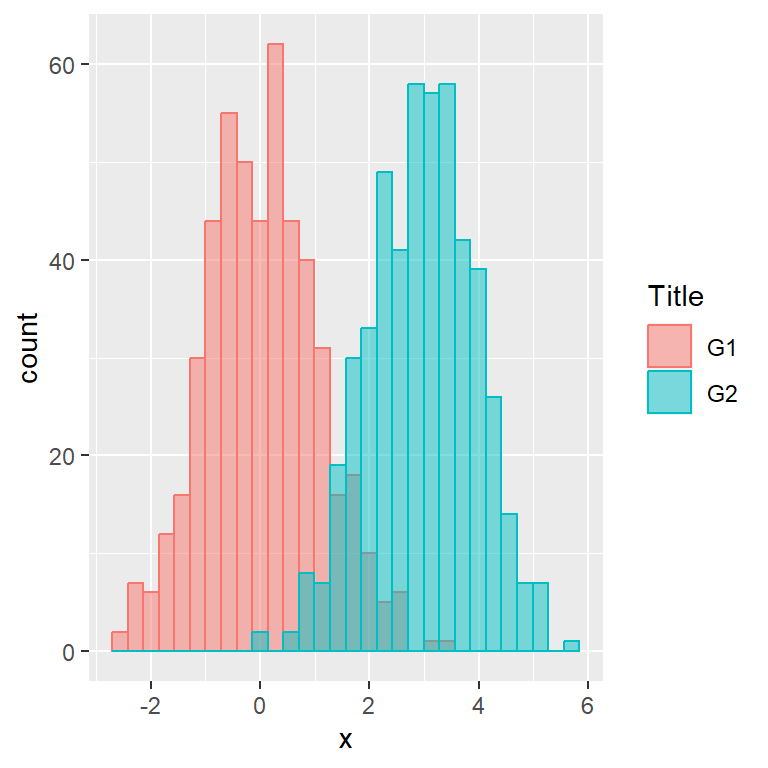Custom legend labels

The legend will display the names of the categorical variable by default, but you can change them with `scale_color_discrete` and/or `scale_fill_discrete`. Note that this will depend to which `aes` you set.

``````# install.packages("ggplot2")
library(ggplot2)

# Histogram by group in ggplot2
ggplot(df, aes(x = x, fill = group, colour = group)) +
geom_histogram(alpha = 0.5, position = "identity") +
scale_color_discrete(labels = c("A", "B")) +
scale_fill_discrete(labels = c("A", "B"))``````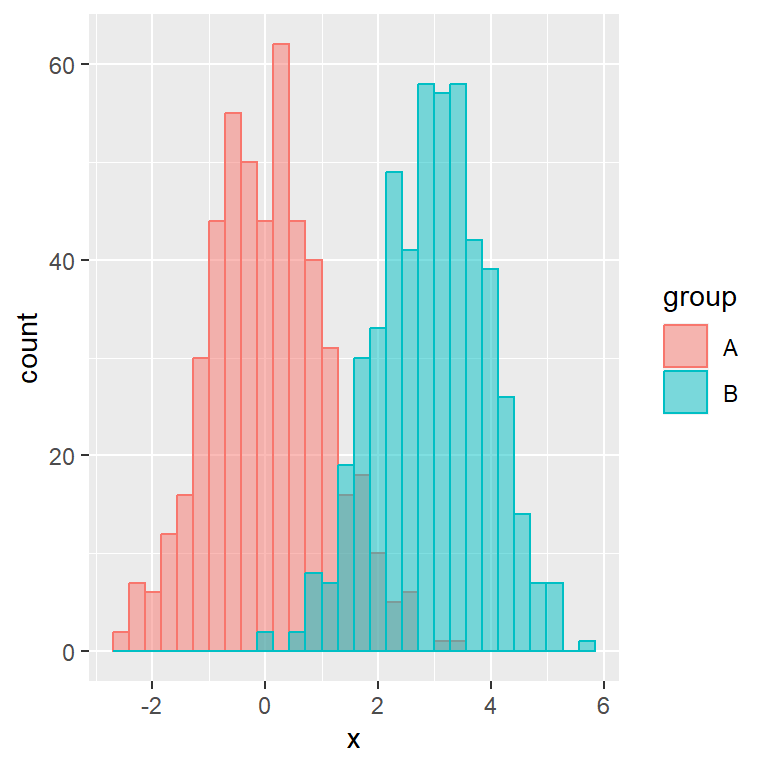Legend position

The position of the legend defaults to the right, but can be changed with the `legend.position` component of the `theme` function as in the example below.

``````# install.packages("ggplot2")
library(ggplot2)

# Histogram by group in ggplot2
ggplot(df, aes(x = x, fill = group, colour = group)) +
geom_histogram(alpha = 0.5, position = "identity") +
theme(legend.position = "left")``````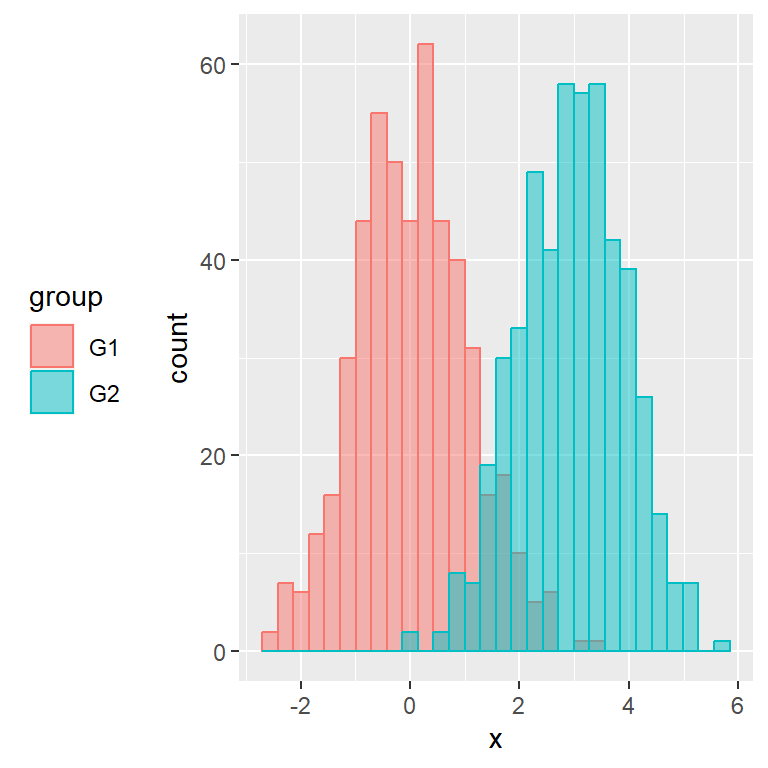Remove the legend

Setting `position = "none"` the legend will be completely removed.

``````# install.packages("ggplot2")
library(ggplot2)

# Histogram by group in ggplot2
ggplot(df, aes(x = x, fill = group, colour = group)) +
geom_histogram(alpha = 0.5, position = "identity") +
theme(legend.position = "none")``````